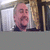## Convert CPU time into MIPSTags:
390
CPU
IBM DB2
Mainframe
How to convert CPU time into MIPS? I would like to convert CPU time of an address space into MIPS. Thanks!

Thanks. We'll let you know when a new response is added.

This makes the assumption that the job is not multi-tasking either through it’s own code or with the built-in cpu parallelism of DB2.

1) divide the cpu time by the elapsed time
2) divide the total processor MIPS by the number of engines on the processor
3) multiply the two above results together

Example:

1) A job that ran for 60 minutes consumed 20 minutes of cpu time. So 20/60 = .33

2) The processor has a 6 engines and a total of 1200 MIPS. So 1200/6 = 200 MIPS per engine.

3) 200*.33 = 66 MIPS

Bill

Hmmmm…. I’m not sure I’m on board with the math here. While I’m okay with the answer, I’m not so okay with Bill’s logic.

Let’s suppose for instance that this same process (which requires 20 minutes of CPU time) actually ran for 100 minutes due to a heavy load on the processor caused by other jobs running. However, the job still only required 20 minutes of CPU time. Now the math because 20/100 = .2, and 200*.2 = 40 MIPS, or

Let’s suppose for instance that this same process (which requires 20 minutes of CPU time) actually ran for 20 minutes due to no load on the processor (i.e. no other processes competing for CPU time). However, the job still only required 20 minutes of CPU time. Now the math because 20/20 = 1.0, and 200*.10 = 200 MIPS.

Obviously the same job can’t require 66, 40, and 200 MIPS on the same machine.

## Discuss This Question: 4 Replies

Thanks. We'll let you know when a new response is added.
••••